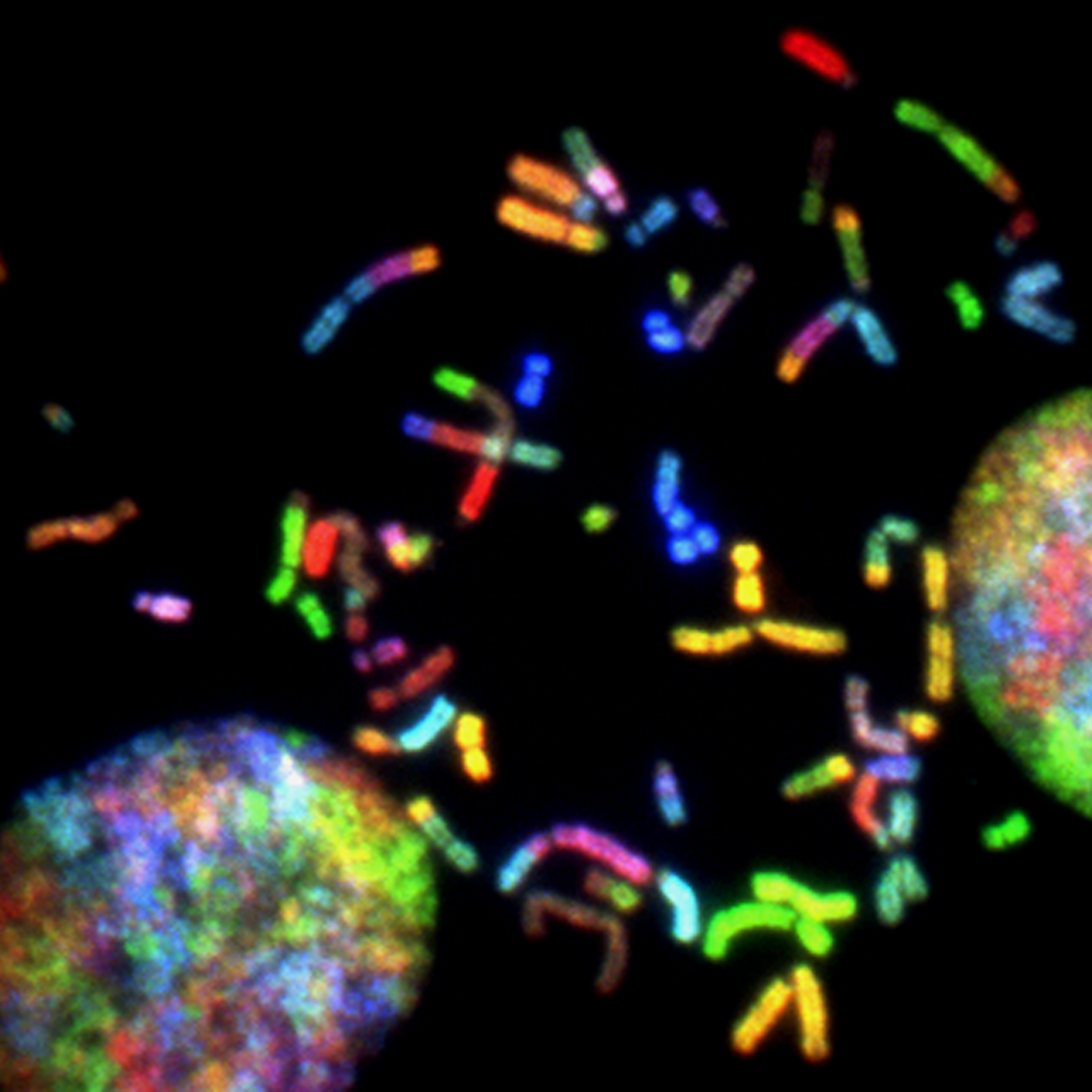# Mahmoud Ahmed

Postdoc - Cancer Genomics

# Introduction to data analysis in R

A gentle introduction to data analysis in R (Summer course)

### Description

This is a short course on data analysis in R. The goal is to get the participants familiar with R code and to appreciate it as a tool to handle common experimental data. The materials are delivered in the form of lectures and interactive exercises.

### Objectives

• To get familiar with R as a tool for data analysis
• To apply basic arithmetic and statistics in R
• To learn to handle experimental data in R (qPCR and images)

### Format

Each module is delivered in three steps

• Lecture: slides discussing the main concepts -Practice: interactive exercises for practicing purposes
• Homework: more exercises to test understanding

### Outline

• Getting started in R
• Lecture
• Basic statistics in R
• Lecture
• Quantifying mRNA using the pcr package
• Lecture
• Quantifying protein co-localization in fluorescence images using the colocr package
• Lecture